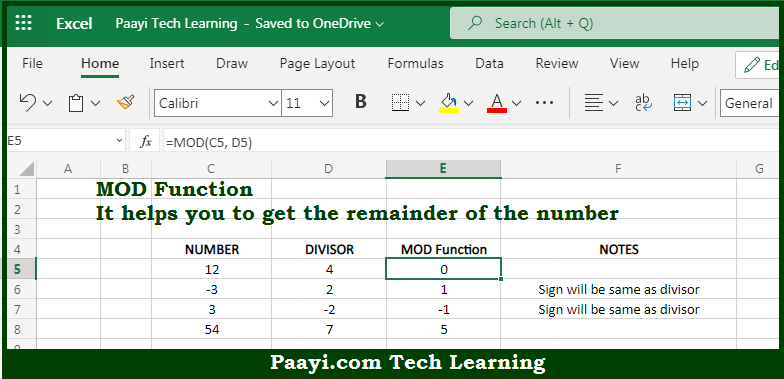# Learn How to Use Microsoft Excel MOD Function

Written by | 0 Comments | 561 Views

In this article, you will learn how to use the Microsoft Excel MOD function and its prime function in Microsoft Excel. You will also get to know the Microsoft Excel MOD function return value and syntax with the help of some examples.

Microsoft Excel MOD Function

The main function of the Microsoft Excel MOD function is to get the remainder from the division. That implies, with the help of MOD function you can able to return the remainder of two numbers after division. Let's say for example MOD(7,2) will return 1. The result of MOD carries the same sign as the divisor. So, with the help of MOD function, you can able to get the remainder from the division.

Return Value of MOD Function

The return value will be the remainder.

Syntax of MOD Function

=MOD(number, divisor)

Where the arguments:

• number: This is the number to be divided with.
• divisor: This is the number to divide with.

## How to Use Microsoft Excel MOD Function?So we know that Microsoft Excel MOD function you can able to get the remainder after division. That implies, with the help of MOD function you can able to return the remainder of two numbers after division. Let's say for example MOD(7,2) will return 1. The result of MOD carries the same sign as the divisor. It should be noted that the MOD function always returns the result in the same sign as the divisor, moreover in case if the divisor is 0, the return value will be #DIV/0! error. So, with the help of MOD function, you can able to get the remainder from the division.